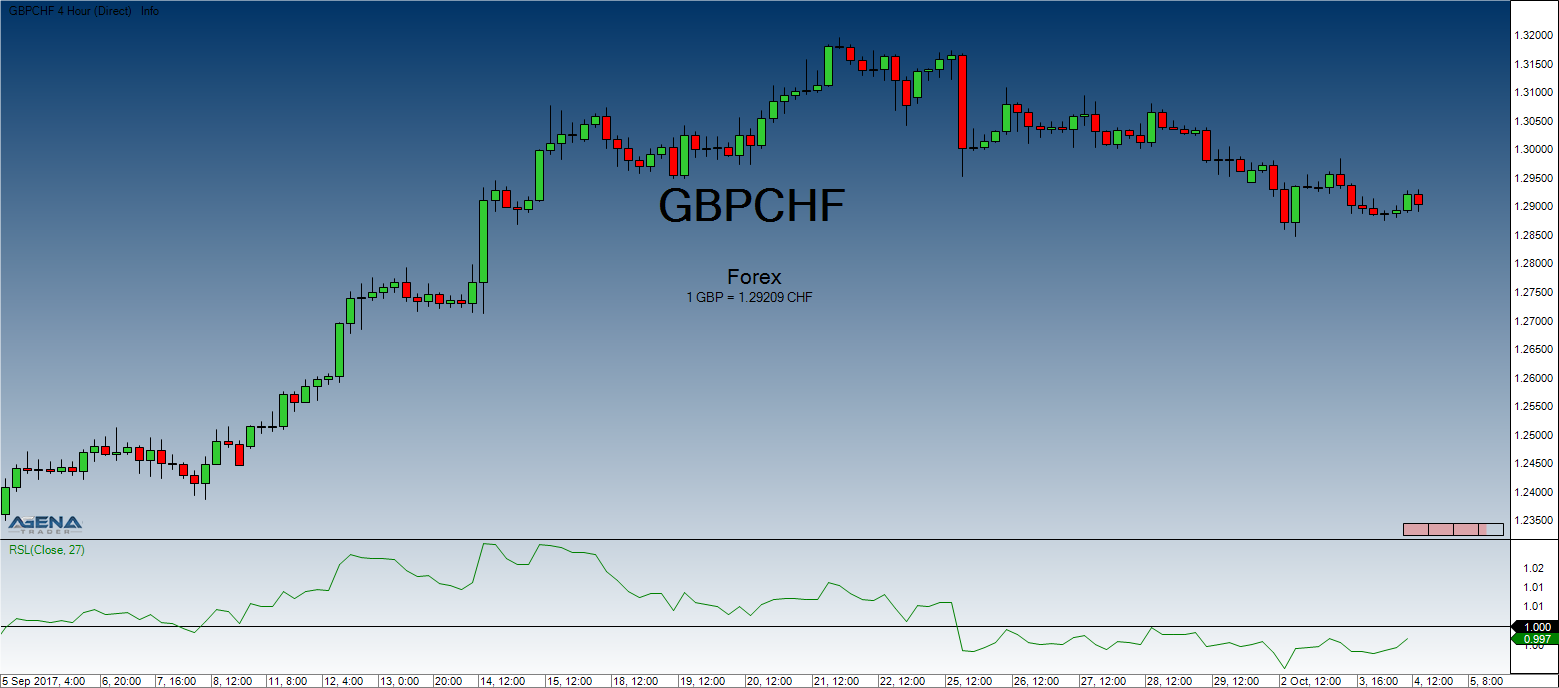# Relative Strength Levy (RSL)

#### Description

The concept of the Relative Strength according to R.A. Levy is that the past developments play a very important role in the strength that will be seen in the future. The current performance is compared to the past performance.
The calculation involves taking the weekly closes (or daily closes) and dividing them by the arithmetic mean of the closing prices for the examined period. The result is then balanced around the 10 marker, and an ordered list is created where the value of the highest RSL fills the first place.

#### Usage

RSL(int period)
RSLDataSeries inSeries, int period)
RSLnt period)[int barsAgo]
RSL(IDataSeries inSeries, int period)[int barsAgo]

#### Return value

double
When using this method with an index (e.g. RSL(27)[int barsAgo] ), the value of the indicator will be issued for the referenced bar.

#### Parameters

inSeries Input data series for the indicator
period Number of bars included in the calculations

#### VisualizationRelative Strength Levy (RSL)

#### Example

//RSL value output
Print("The Relative Strength as calculated by Levy is " + RSL(27));